# How to Analyze Financial Health of a Company in 6 Easy Steps

Financial health analysis (FHA), as the word says, is to analyze the financial health of a company.

The analysis is primarily performed by the management of companies to assess the business sustainability of its suppliers. The overall health of a supplier sheds light on how well it will do in the short term and whether it will be able to honor the supply agreements.

For example, Apple has a business agreement with 320 suppliers.

At periodic intervals, the financial manager of product sourcing (procurement) team checks the financial health of its suppliers to assess whether a supplier would be able to honor the supply agreement of the products. The company also analyzes the financial health while entering into an agreement with a new supplier.

Financial health analysis is also now done by independent Supplier Assessment companies.

The Financial Analyst in these companies analyzes the financial health for the suppliers of a company (such as Apple) and provides the analysis to the financial manager, who can take decision for existing or new supply agreement.

So, how does a financial manager or financial analyst analyze the financial health of a company?

Here's the process:

The financial health can be analyzed by understanding the financial performance with respect to industry performance.

However, looking at large sets of financial data for the company and its peers can be overwhelming.

Also, taking a strategic decision for a supply agreement just based on the looking at the financial performance could be difficult.

Hence, we will prepare a simple model to deduce a score to depict the financial health of a company.

The model will help you to understand the process carried out in calculating the score, which you can also compare historically to deduce the improvement or deterioration in the financial health of the company.

For example, if a supplier score has deteriorated over the period and has entered into a red zone (say less than 30%), the company can negotiate the terms with this supplier.

Similarly, you can look at the score of a supplier, with respect to the other suppliers, while entering into the new contract.

The assessment of financial health can be done by analyzing the performance under four broad categories:

1. Profitability
2. Cash Flow
3. Liquidity
4. Leverage

We will calculate a score of these categories after analyzing the financial ratios under each of these categories.

In our article, we will take the case example of Cisco Systems. We will calculate the Peer benchmark score, which will help understand the financial health of Cisco relative to its peers. We have chosen the following peers of Cisco for our analysis: Motorola Solutions, Juniper Networks, Brocade Communications, and Finisar Corporation.

The below steps will help to calculate the aggregate score to assess financial health.

How to Analyze Financial Health of a Company in 6 Easy Steps

## 1. Prepare the data

The financial data to analyze the financial ratios can be sourced from the filings of a company.

Please source the following financial items from the income statement, balance sheet and cash flow statement of the financial statement. For the purpose of ratio calculation, source 2 years of historical data for each of the peers.

Figure 1: Historical data for Health analysis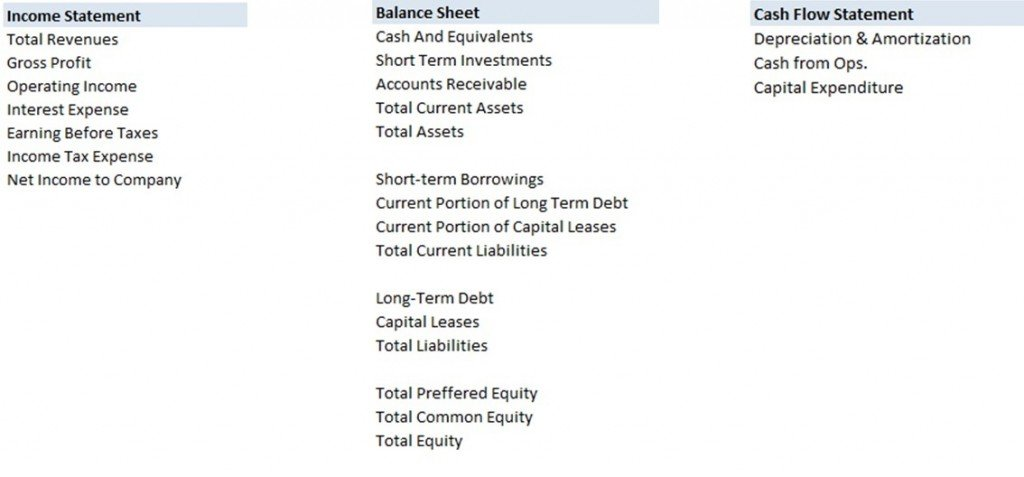## 2. Calculate Profitability Score

The profitability of the company can be analyzed by calculating the following ratios for the company and its peers.

### a) Gross Margin

This ratio indicates how profitable a company is at the most fundamental level and is calculated as:

= Gross Profit / Revenues

### b) EBITDA Margin

This ratio indicates what proportion of a company's revenue is left over after paying for variable costs of production such as wages, raw materials, etc. and is calculated as:

= EBITDA / Revenues

### c) Net Profit Margin

This ratio Indicates how much profit a company makes for every dollar it generates in revenue and is calculated as:

= Net Profit / Revenues

### d) Return on Assets

This ratio indicates how efficiently the management using its assets to generate earnings and is calculates as:

= EBIT x (1-Tax Rate) / Total Assets

Once the ratios are calculated for Cisco and its peers then set up the table as shown in Figure 2 to calculate the Profitability Score.

In order to calculate the Profitability Score, we will first assign the weight to each of the ratios and then calculate the score for each of the ratios.

We will then multiply the weight and score of each ratio and add them to calculate to the Profitability Score.

### Weight Assumptions

The weight of a ratio is assigned by understanding the relevance of that ratio relative to its peers.

The idea is that the ratio and the financial (used to calculate that ratio) should be comparable across the companies.

If the financial item is not comparable, you should try to adjust for unusual or extraordinary items to make it comparable and then calculate the ratio for comparison purpose. If you are not able to make the desired adjustment (due to lack of disclosures) then either choose a different ratio or assign that ratio low or nil weight.

For example, if the net profit of the company (that you are analyzing) is negative and of all peers is positive, then you should find the reason for negative profit.

If the disclosures are available, then you should adjust the net profit to make it comparable. If the disclosures are not available, then either choose a difference ratio (such as EBIT Margin) or assign Net Profit Margin 0% weight and distribute the weights to other ratios.

Similarly, if the company that you are analyzing has less debt on its balance sheet as compared to its peers, then the leverage position or debt ratios will not be comparable. Hence, assign less weight to the leverage category and debt ratios (for example, Total Debt to Equity) and coverage ratios (Interest coverage).

For our example of Cisco in Figure 2, we have assigned less weight to EBITDA margin and Return on Assets, because the ratios are very near to the boundaries (minimum and maximum) of peer average.

We will follow the same approach of assigning weights when we calculate the ratios in other categories.

### Score Calculation

We will now calculate the score for each ratio.

In order to calculate the score, we will first calculate the minimum and maximum of each ratio of all peers (including Cisco). Then we will calculate the score of Cisco on a scale of 0 to 10 (0 being the lowest and 10 being the highest). This process is called normalization.

To understand normalization, consider that you need to find the position of a number between lower boundary and an upper boundary. For example, 8 is at the 8th position between 0 and 10 (with 0 being the minimum and 10 being the highest).  This can be calculated as (8 – 0)/(10 – 0).

We will use this concept to calculate the score of each ratio.

For example, Gross Margin of Cisco is 60.3% and a minimum of peers is 34.8% and a maximum of peers is 66.3%.

The normalized score of Gross Margin (on a scale of 0 to 10) can be calculated as [(60.3% – 34.8%) / (66.3% – 34.8%)] * 10. Please note that 0 is the lowest score and 10 is the highest score.

Once, you have set up the weights and calculated score for each ratio, you can calculate the profitability score by summing up the multiplication of weight and score of each ratio. This is done using the Sumproduct function of Excel.

In practice, you should assign the weight for a financial ratio based on an understanding of the company. This is the approach followed by a financial manager or financial analysts.

Also, you should exclude the outliers (companies having very high or low ratios) from the peer set or calculation of minimum and maximum. In practice, the minimum and maximum are also calculated by taking the full data set of companies in a broad index, like the S&P 500.

Figure 2: Calculation of Profitability Score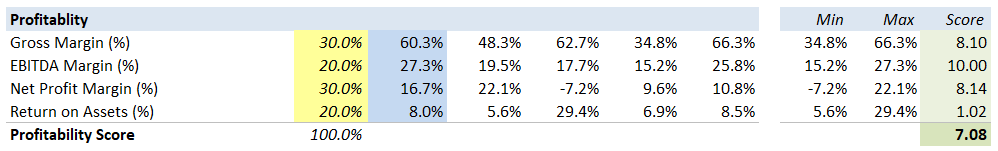## 3. Calculate Liquidity Score

The liquidity of the company can be analyzed by calculating the following ratios for the company and industry peers.

### a) Current Ratio

This ratio indicates the firm’s ability to meet short-term obligations from short-term assets employed in the firm and is calculated as:

= Current Assets / Current Liabilities

### b) Quick Ratio

This ratio indicates the firm’s ability to meet short-term obligation from short-term liquid obligations employed in the firm and is calculated as:

= (Cash and Short term investment) / Current Liabilities

### c) EBITDA / Interest Expense

This ratio indicates the firm’s ability to meet the interest expense from earning generated by the business and is calculated as:

= EBITDA / Interest Expense

Where EBITDA is calculated as EBIT + Depreciation + Amortization

### d) CFO / Current Debt

This ratio indicates the firm’s ability to meet the current debt obligation from cash generated by the business and is calculated as:

= Cash Flow from Operation / Short Term Borrowings

We will apply a similar approach to calculate the liquidity score as we used to calculate profitability score.

Figure 3: Calculation of Liquidity Score

The full calculation of Liquidity score for CISCO Corp can be seen in the spreadsheet here.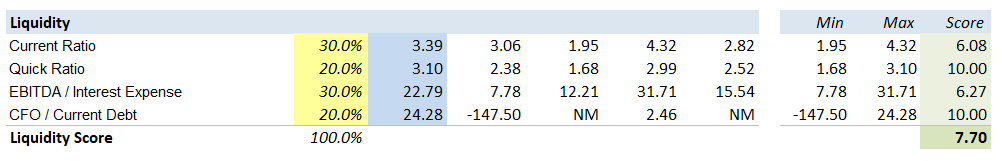## 4. Calculate Cash Flow Score

The cash flow of the company can be analyzed by calculating the following ratios for the company and industry peers.

### a) Accounts Receivable Turnover

The ratio indicates the effectiveness of the company’s credit control department in collecting the outstanding money and is calculated as:

= Total Revenue / Average Receivables

### b) CFO / Current Liabilities

This ratio indicates the firm’s ability to meet short-term obligations from the cash flows (from operations) generated by the business and is calculated as:

= Cash Flow from Operations / Current Liabilities

### C) Discretionary Cash Flow

This ratio indicates the cash generated by the business after laying out the money required to maintain or expand its asset base and is calculated as:

= EBIT x (1-Tax Rate) + Depreciation & Amortization - Change in Net Working Capital - Capital Expenditure

We will apply a similar approach to calculate the Cash Flow score as we used to calculate profitability score.

Figure 4: Calculation of Cash Flow Score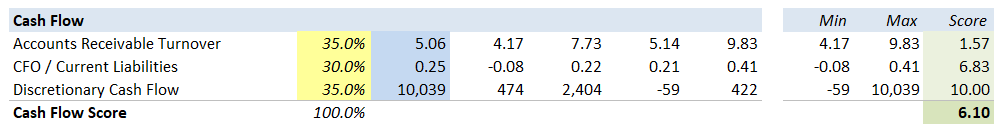## 5. Calculate Leverage Score

The leverage/capital structure of the company can be analyzed by calculating the following ratios for the company and industry peers.

### a) Total Debt / Equity

This ratio indicates the proportion of debt capital with respect to equity capital employed in the business and can be calculated as:

= Total Debt / Total Equity

where Total Debt = Short Term Borrowing + Total Long Term Borrowing + Capital Leases and

Total Equity = Total Preferred Equity + Total Shareholder’s Equity

### b) Total Debt / Capital

This ratio indicates the proportion of debt capital with respect to total capital employed in the business and can be calculated as:

= Total Debt / (Total Debt + Total Equity)

### c) Total Liabilities / Total Assets

This ratio indicates the proportion of total liabilities with respect to total assets in the business and can be calculated as:

= Total Liabilities / Total Assets

### d) Total Debt / EBITDA

This ratio indicates the repaying capacity of the borrowers with the cash income generated by the business and is calculated as:

= Total Debt / Total EBITDA

We will apply a similar approach to calculate the leverage score as we used to calculate profitability score.

Figure 5: Calculation of Leverage Score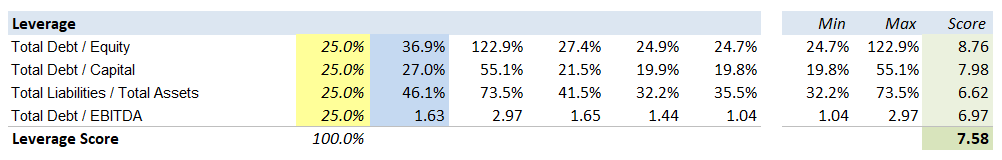## 6. Calculate Aggregate Score

We will now assign the weight to each of the broad ratio category scores to calculate the aggregate score for peer benchmark and historical benchmark.

The consolidated company score can be calculated by the Sumproduct of weight and score.

Figure 6: Calculation of Aggregate Score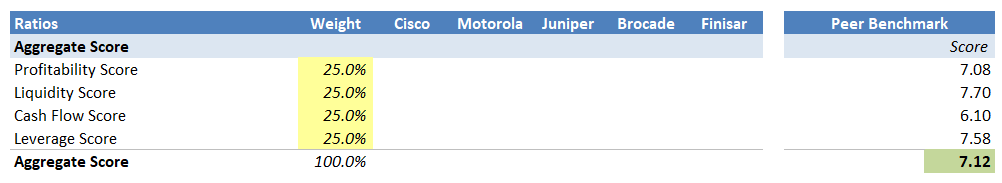Based on the above calculations, we can observe that Cisco has a strong profitability position, supported by a large revenue base and healthy margins.

The liquidity position is strong, marked by a substantial cash reserve and a high current ratio.

The company generated enough earnings and cash flows to cover up the interest and debt obligations.

The leverage position is strong, supported by a low net debt level and healthy coverage of liabilities.

Suggested: Read more about Financial Modeling Examples

### Key Takeaway

The aggregate score was above 70%, which places Cisco in strong position amongst its peers for supply assessment. The financial manager can compare the score historically to see the improvement/deterioration in financial health.

## Now It’s Your Turn

You’ve just seen 6 of my favorite Financial Health Analysis secrets…

Now it’s time to put these techniques into practice.

The first step?

Enter your email to get access to my FREE Financial Modeling Videos and eBooks.

The next step?

Write your comments and queries below.

Next Classroom Batches in India
- Classroom Course with Placement Assistance: Our Full-Time Financial Modeling Investment Banking Course (6 Weeks) starts on 16 September, 2019 and 2.5 Months Weekend Workshop starts on 27 September in New Delhi, India and on 16 September, 2019 in Gurgaon, India.

- Online Course with Placement Assistance: Join our Online Financial Modeling Investment Banking course with Placement Assistance from anywhere in the world.

- CFA Level - I: 16th November, 2019

- Big Data: 29th June, 2019

- Financial Modeling Investment Banking Weekend in Gurgaon: 27 September, 2019

- See Testimonials.

Only a few seats remain. Interested candidates can contact me.

### 8 thoughts on “How to Analyze Financial Health of a Company in 6 Easy Steps”

1.Great help thanks

2.Hi, thank you so much for this, you explain on a wway that make it easier to understand the full process.

I only missed the figure for the item 3 3. Calculate Liquidity Score.

would you mind to replace it?

thanks!

•Yes..
Figure 3 is same with Figure 2.
would you mind to replace it?

Thanks

•Hi Naz,

Thank you for pointing out. Just updated. Please check.

3.Hi, I am a Chartered Accountant and pass out in may 2013. Want to know more about this course and job placement assistance provided by you. I really want to persue this if it fullfills my aspirations.
Thanks

•Hi Ankit,

Please email me ([email protected]) your conatact number. My associate will call you to provide complete program details.

4.Thanks for sharing it. This is really good and informational.

•Thanks Deepti, implement and share your results with me 🙂